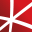# Top 20 NuGet algebra Packages

Math.NET Numerics is the numerical foundation of the Math.NET project, aiming to provide methods and algorithms for numerical computations in science, engineering and every day use. Supports .Net Framework 4.0 or higher and .Net Standard 1.3 or higher, on Windows, Linux and Mac.
Math.NET Symbolics is a basic open source computer algebra library for .Net and Mono. Written in F# but works well in C# as well. Supports .Net Framework 4.5 or higher and .Net Standard 2.0 or higher, on Windows, Linux and Mac.
The Extreme Optimization Numerical Libraries for .NET are a set of libraries for numerical computing and data analysis. This is the main package that contains all the core functionality. For optimal performance, we strongly recommend also referencing one of the native packages based on Intel's Mat...
F# Modules for Math.NET Numerics, the numerical foundation of the Math.NET project, aiming to provide methods and algorithms for numerical computations in science, engineering and every day use. Supports .Net Framework 4.5 or higher and .Net Standard 1.6 or higher, on Windows, Linux and Mac.
Math.NET Numerics is the numerical foundation of the Math.NET project, aiming to provide methods and algorithms for numerical computations in science, engineering and every day use. Supports .Net Framework 4.0 or higher and .Net Standard 1.3 or higher, on Windows, Linux and Mac. This package contain...
Foundational classes for financial, engineering, and scientific applications, including complex number classes, general vector and matrix classes, structured sparse matrix classes and factorizations, general sparse matrix classes and factorizations, general matrix decompositions, least squares solut...## Math.NET Numerics - MKL Native Provider for Windows (x64)

Intel MKL native libraries for Math.NET Numerics on Windows.
Foundational classes for financial, engineering, and scientific applications, including complex number classes, general vector and matrix classes, structured sparse matrix classes and factorizations, general sparse matrix classes and factorizations, general matrix decompositions, least squares solut...## NMath - Standard Library - OSX x64 (alpha)

Foundational classes for financial, engineering, and scientific applications, including complex number classes, general vector and matrix classes, structured sparse matrix classes and factorizations, general sparse matrix classes and factorizations, general matrix decompositions, least squares solut...## NMath - Standard Library - Windows and Linux - x64

Foundational classes for financial, engineering, and scientific applications, including complex number classes, general vector and matrix classes, structured sparse matrix classes and factorizations, general sparse matrix classes and factorizations, general matrix decompositions, least squares solut...## NMath - Standard Library - Windows - x86 and x64

Foundational classes for financial, engineering, and scientific applications, including complex number classes, general vector and matrix classes, structured sparse matrix classes and factorizations, general sparse matrix classes and factorizations, general matrix decompositions, least squares solut...
Foundational classes for financial, engineering, and scientific applications, including complex number classes, general vector and matrix classes, structured sparse matrix classes and factorizations, general sparse matrix classes and factorizations, general matrix decompositions, least squares solut...
Contains a matrix extension library, along with a suite of numerical matrix decomposition methods, numerical optimization algorithms for constrained and unconstrained problems, special functions and other tools for scientific applications. This package is part of the Accord.NET Framework.
Function programming goodness: algebraic structures, Maybe, Either, Unit, State, Writer, Functor, Monad, Monoid, and more.
A simple cross platform .NET API for Intel MKL. Reference the MKL.NET package and required runtime packages and use the static MKL functions. The correct native libraries will be included and loaded at runtime. Exposing functions from MKL keeping the syntax as close to the c developer reference as...
A simple cross platform .NET API for Intel MKL. Reference the MKL.NET package and required runtime packages and use the static MKL functions. The correct native libraries will be included and loaded at runtime. Exposing functions from MKL keeping the syntax as close to the c developer reference as...
A simple cross platform .NET API for Intel MKL. Reference the MKL.NET package and required runtime packages and use the static MKL functions. The correct native libraries will be included and loaded at runtime. Exposing functions from MKL keeping the syntax as close to the c developer reference as...
A simple cross platform .NET API for Intel MKL. Reference the MKL.NET package and required runtime packages and use the static MKL functions. The correct native libraries will be included and loaded at runtime. Exposing functions from MKL keeping the syntax as close to the c developer reference as...
A simple cross platform .NET API for Intel MKL. Reference the MKL.NET package and required runtime packages and use the static MKL functions. The correct native libraries will be included and loaded at runtime. Exposing functions from MKL keeping the syntax as close to the c developer reference as...
A simple cross platform .NET API for Intel MKL. Reference the MKL.NET package and required runtime packages and use the static MKL functions. The correct native libraries will be included and loaded at runtime. Exposing functions from MKL keeping the syntax as close to the c developer reference as...